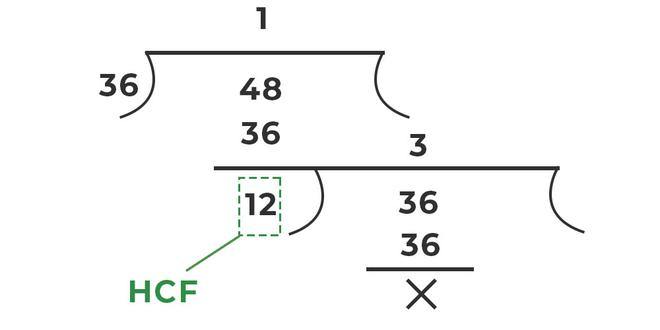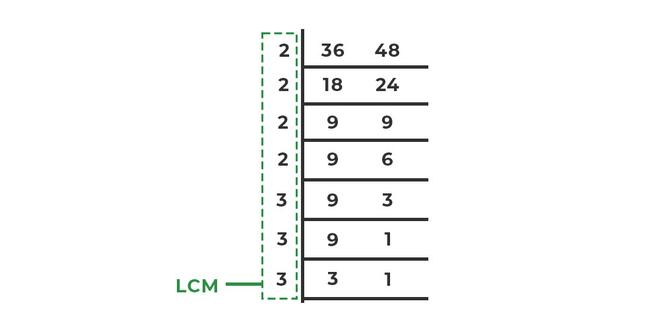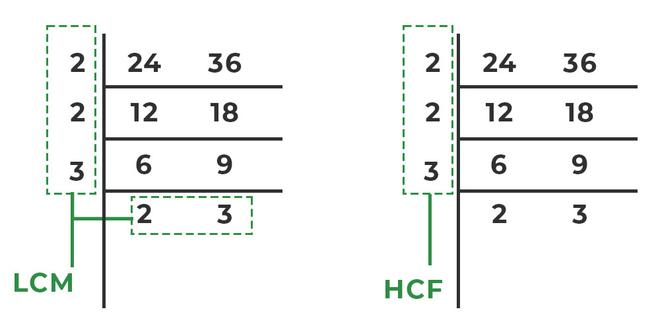Open in App
Not now

# HCF and LCM

• Difficulty Level : Basic
• Last Updated : 10 Aug, 2022

The terms HCF and LCM full forms are the Highest common factor and Least Common Multiple. HCF (Highest Common Factor) is used for two or more numbers to get the greatest number which divides all the given numbers without getting any remainder. That is why HCF is also known as the greatest common divisor. LCM (Least Common Multiple) is used for two or more two numbers to get the least positive number which gets divided by all the given numbers without leaving any remainder.

## What are HCF and LCM?

HCF is the Highest Common Factor which can be seen in two or more numbers. HCF is the greatest common number that divides all the given numbers exactly. Therefore, HCF is also known as Greatest Common Divisor, also known as GCD. For example, If we take 13 and 91, then HCF will be 7 as 7 is the highest common factor which will divide both 13 and 91 without leaving any remainder. LCM is the Least Common Multiple that can be seen in two or more numbers. LCM is the least number that is a common multiple of all the given numbers. For example, If we take 3 and 7 then 21 will be the LCM as 21 is the least common multiple, which will be divided by 3 and 7 without leaving any remainder. Let’s dig deeper and find out how to find HCF and LCM.

## How to Find HCF and LCM?

There are various methods to find HCF and LCM. Following are some of the most famous methods used to calculate the Highest Common factor and Least Common Multiple.

1. Division method
2. Factorization method
3. Prime factorization method

### HCF by Division Method

The easiest way to understand how to find HCF by Division Method is by going back to simple division. Following are the steps for better understanding,

Step 1: Take the smaller number as the divisor and the larger number as a dividend.

Step 2: Perform division. If you get the remainder as 0, then the divisor is the HCF of the given numbers.

Step 3: If you get a remainder other than 0 then take the remainder as the new divisor and the previous divisor as the new dividend.

Step 4: Perform steps 2 and step 3 until you get the remainder as 0.

Example: Find out the HCF of 36 and 48.

Solution:

Using the division method for HCFHence, HCF = 12

### LCM by Division Method

In order to find LCM formula by Division method, we divide the Following steps can be followed in order to find Least Common Division by Division Method:

Step 1: Check whether the given numbers are divisible by 2 or not.

Step 2: If the number is divisible by 2 then divide and again check for the same. If the numbers are not divisible by 2 then check 3, and so on.

Step 3: Perform step 2 until you get 1 in the end.

Example: Find out the LCM of 36 and 48.

Solution:

Using the division method for LCMHence, LCM = 2 × 2 × 2 × 2 × 3 × 3 × 3 = 432

### HCF by Factorization Method

Finding HCF by Factorization method requires the given steps to be followed:

Step 1: Write all the divisors of the given number.

Step 2: Check for common divisors among them and find the greatest common divisor. This greatest common divisor will be the HCF of the given numbers.

Example: Find out the HCF of 6 and 18.

Solution:

Divisors of 6 = 1, 2, 3, 6

Divisors of 18 = 1, 2, 3, 6, 9, 18

HCF = greatest common divisor

HCF = 6

### LCM by Factorization Method

The factorization method for LCM requires minimum steps; below are the steps mentioned:

Step 1: Write the multiples of the given numbers until you reach the first common multiple.

Step 2: First common multiple of the given numbers will be the LCM.

Example: Find out the LCM of 6 and 18.

Solution:

Multiple of 6 = 6, 12, 18, 24, 30, …

Multiple of 18 = 18, 36, 54, …

LCM = first common multiple (least common multiple)

LCM = 18

### HCF by Prime Factorization

Finding HCF by Prime Factorization can be done by following the given steps:

Step 1: Find out the prime factors of the given number.

Step 2: Check the occurrence of a particular factor. Find out the common factors and choose them in HCF.

Step 3: Multiply the occurrence of common factors. And this will be the HCF Of the given numbers.

Example: Find out the HCF of 18 and 90.

Prime factors of 18 = 2 × 3 × 3

Prime factors of 90 = 2 × 3 × 3 × 5

Now, HCF = 2 × 3 × 3 = 18

### LCM by Prime Factorization

Finding LCM by Prime Factorization is done by following the given steps:

Step 1: Find out the prime factors of the given number.

Step 2: Check the occurrence of a particular factor. If a particular factor has occurred multiple times in the given number, then choose the maximum occurrence of the factor in LCM. It can also be found out by checking the powers of the factors. The factor having greater power will be chosen between the numbers.

Step 3: Multiply all the maximum occurrences of a particular factor. And this will be the LCM Of the given numbers.

Example: Find out the LCM of 18 and 90.

Solution:

Prime factors of 18 = 2 × 3 × 3

Prime factors of 90 = 2 × 3 × 3 × 5

Now, LCM = 2 × 3 × 3 × 5 = 90

Alternate method:

Prime factors of 18 = 2 × 3 × 3

Prime factors of 18 = 21 × 32

Prime factors of 90 = 2 × 3 × 3 × 5

Prime factors of 90 = 21 × 32 × 51

Chosen factors for LCM = 21 × 32 × 51

Therefore, LCM = 2 × 9 × 5 = 90.

### HCF and LCM Formula

In order to find the HCF and LCM formula, let’s assume that the numbers given are a and b. The relationship between HCF and LCM states that the product of a and b is equal to the product of HCF and LCM.

(LCM of two numbers) × (HCF of two numbers) = Product of two numbers

Mathematically this can be written as:

LCM(a, b) × HCF(a, b) = a × b

## Solved Examples on HCF and LCM

Example 1: Find out the LCM and HCF of 18, 30, and 90 by prime factorization.

Solution:

Prime factors of 18 = 2 × 3 × 3

Prime factors of 30 = 2 × 3 × 5

Prime factors of 90 = 2 × 3 × 3 × 5

LCM: 2 × 3 × 3 × 5 = 90

HCF: 2 × 3 = 6

Example 2: Find out the LCM and HCF of 318 and 504.

Solution:

Prime factors of 16 = 2 × 2 × 2 × 2

Prime factors of 30 = 2 × 3 × 5

LCM: 2 × 2 × 2 × 2 × 3 × 5

HCF: 2

Example 3: Find out the HCF and LCM of 24 and 36.

Solution:

Let’s find out the HCF and LCM together by division method,Therefore,

HCF = 2 × 2 × 3 = 12

LCM = 2 × 2 × 3 × 2 × 3 = 72

Example 4: Find out the LCM and HCF of 15 and 70. Also, verify the relationship between LCM, HCF, And given numbers.

Solution:

Prime factors of 15 = 3 × 5

Prime factors of 70 = 2 × 5 × 7

LCM: 2 × 3 × 5 × 7

HCF: 5

Verifying the relationship:

LCM × HCF = 2 × 3 × 5 × 5 × 7 = 1050

Product of two numbers = 15 × 70 = 1050

From above you can see that

LCM (15, 70) ×  HCF(15, 70) = Product of two numbers

Hence Verified.

## FAQs on HCF and LCM

Question 1: State the full form of HCF and LCM.

The full form of HCF is the Highest Common Factor and the full form of LCM is the Lowest Common Multiple. For example, the HCF of 4 and 12 is 4, the LCM of 3 and 10 is 30.

Question 2: What is the relationship between HCF and LCM?

The relationship between HCF and LCM states that the product of a and b is equal to the product of HCF and LCM.

(LCM of two numbers) × (HCF of two numbers) = Product of two numbers

This is written as:

LCM(a, b) × HCF(a, b) = a × b

Question 3: What is GCF? Find GCF of 4 and 12.

GCF is the Greatest Common Factor which is nothing but another name for HCF.

GCF of 4 and 12 will be 4 as 4 is the greatest common factor between 4 and 12.

Question 4: What are the different methods to find HCF and LCM in math?

There are various methods to find HCF and LCM. Following are some of the most famous methods used to calculate the Highest Common factor and Least Common Multiple.

1. Division method
2. Factorization method
3. Prime factorization method

Question 5: What is the use of HCF and LCM?

HCF is very useful in maths and in real life as well. When numbers are required to be divided into smaller sections, or when certain things are required to be into smaller parts or in groups, HCF is used. LCM can be used in places where certain situations will require to occur multiple times.

### Related Resources

CBSE Class 8 Maths Notes for Factorization

CBSE Class 10 Maths Notes for Factorization

My Personal Notes arrow_drop_up
Related Articles